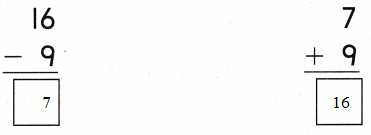Refer to our Texas Go Math Grade 1 Answer Key Pdf to score good marks in the exams. Test yourself by practicing the problems from Texas Go Math Grade 1 Module 13 Assessment Answer Key.

Vocabulary

Question 1.
Complete the related facts. (p. 460)
9 + ________ = 15
6 + _________ = 15
15 – _________ = 9
15 – _________ = 6
9 + 6 = 15
6 + 9 = 15
15 – 6 = 9
15 – 9 = 6

Concepts and Skills

Question 2.
Complete the related facts (TEKS 1.5.F, 1.5.G)
________ + 8 = 17
8 + 9 = __________

17 – ________ = 9
_________ – ____________ = ___________
9 + 8 = 17
8 + 9 = 17
17 – 8 = 9
17 – 9 = 8

Question 3.Question 4.
Write the unknown numbers. Explain how you got your answer. (TEKS 1.5.F, 1.S.G)
________ + 5 = 13
13 – 5 = __________
8 + 5 = 13
13 – 5 = 8

Question 5.
Which number will show expressions of the same value? (TEKS 1.5.E)
0 + 9 = _________ – 9
(A) 18
(B) 9
(C) 0
18 – 9 = 9
18 will show expressions of the same value.
Thus the correct answer is option A.

Question 6.
Which is the related fact for 9 + 5 = 14? (TEKS 1.5.F, 1.5.G)
(A) 14 – 5 = 9
(B) 9 – 5 = 4
(C) 14 – 4 = 10
9 + 5 = 14
14 – 5 = 9

Question 7.
Which addition fact can you use to check the subtraction for 15 – 6 = ________? (TEKS 1.5.F, 1.5.G)
(A) 5 + __________ = 15
(B) 6 + __________ = 15
(C) 10 = __________ = 15
15 – 6 = 9
6 + 9 = 15
The correct answer is option B.

Question 8.
Which number completes the related fact? 9 + 9 = ________ and ________ – 9 = 9 (TEKS 1.5.F, 1.5.G)
(A) 19
(B) 16
(C) 18
9 + 9 = 18
18 – 9 = 9
Thus the answer is 18 that is option C.

Question 9.
Which expressions have an equal value? (TEKS 1.5.E)
(A) 5 + 12 = 12 – 5
(B) 2 + 10 = 7 + 5
(C) 18 – 7 = 11 + 7